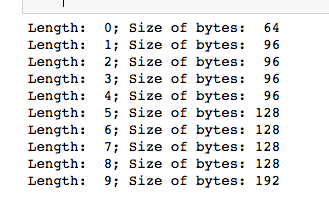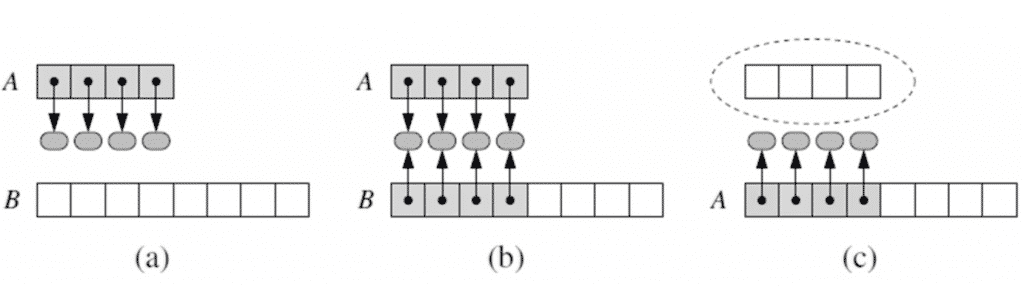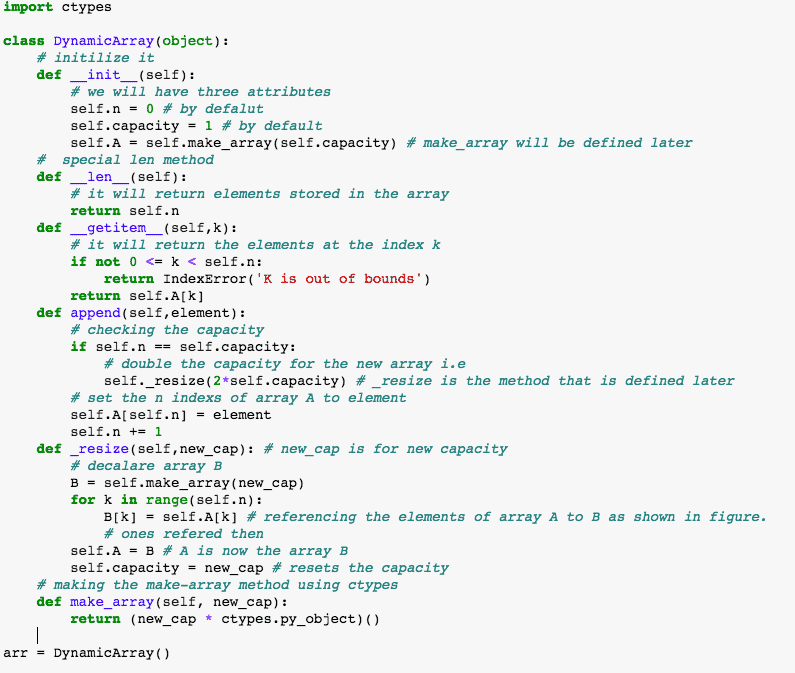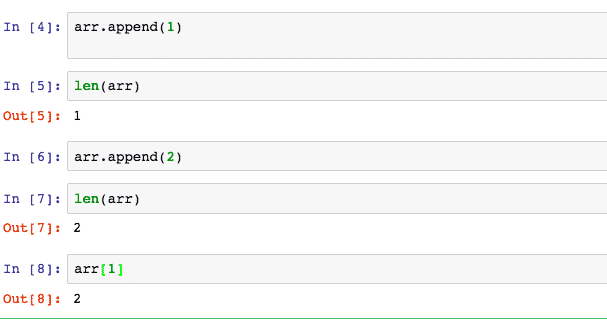Programming

# Implementation of Dynamic Arrays in Python Programming

Arrays can be of static and dynamic types. In this article, we will be focusing on what is a Dynamic Array? and implement it practically through code using the Python programming language.

### What is a Dynamic Array?

In computer science, an array, in general, is a data type that can store multiple values without constructing multiple variables with a certain index specifying each item in them and is used in almost all programming languages like C, Java, C++, C#, Swift etc but, Python has an extra edge over them. When you build a list over Python, then you may have noticed that you really don’t need to specify how large the array or list is beforehand, instead, in python, the list is of dynamic nature i.e. we could just keep adding the item constantly.

So, How does Python actually do this?

Well, the answer is dynamic arrays. Suppose you have a list, the list instance often has the greater capacity than the current i.e if it has 4 or 5 elements then it can store much more than that but if you keep appending the list then, eventually that extra space will run out.

### Basics Behind the Memory Allocation of Array in Python

So, let us see this extra space demonstration by coding practice and relationship between the actual size of the array held in the memory and the given size.

Head over to the Jupiter notebook for the practice. if you don’t have one then download it from here or you can use any editor or development environment of your own choice. Copy the code written below.
``` # Import sys that will allow us to use a get sizeof function which will help us to know the actual size in bytes that computer is holding in the memory.```

import sys
#set n
n = 10
# set empty list
data = []
for i in range(n):

# number of elements
a = len(data)
#actual size of bytes
b = sys.getsizeof(data)
print (‘Length:{0:3d}; Size of bytes:{1:4d}’.format(a, b))
#Increase length by one so
data.append(n)

Note: keep Indentation in mind

Run the code you will see this kind of result as shown in the figure.Now, here is the interesting thing that happens, as we increase the length of the list, bytes also increases but, python does this in chunks so, if the value of n would be 50 then the result would be something like this.i.e python is actually allocating the memory in chunks for different length sizes thus it is intelligent enough to know that when it needs extra space for data allocation then it gives them extra size as shown in the figure.

### Dynamic Array Implementation(Theory)

The purpose is to grow or append a given array or list say that stores the elements of the list and as we know that the array is going to have a fixed capacity so, we just can grow that array.

So, if a list is appended when the size of the array is full then, we need to perform the following steps i.e the algorithm behind the dynamic array implementation.

• Allocate a new array B with a larger capacity
• Set B[i] = A[i], for i=0,….,n-1, where n denotes the current number of the item.
• Set A = B, as now B is referencing our new list.
• And finally, Insert the new element in the new array

An illustration of the above steps through the diagram.

(a) create new array B. (b) store elements of A in B. (c) reassign the reference of items of A in BNow, you just want to know Of what size the new array should be created? right so, the general rule says that the newly created array should be twice of the original array.

### Implementing Dynamic Array(Code)

Here, I will show you a simple example, how to implement this concept in programming practice and day to day problems. Head over to your code editor and copy the codes written below.

We will create our own Dynamic array class. We will be using a built-in library called ctypes which is going to be used as a raw array from the ctypes module.

Also, keep one thing in the note that I have used ‘_’ before methods that I have made just to keep them non-public for Jupiter notebook.Now, as our dynamic array class is ready to let us do some stuff with this. So,So, we have now implemented our dynamic array and that’s it, we can append the array that is a list, in this case, we can also see the value at the index as shown in the above figure.

Try to implement the above demonstration with sys.getsizeof method discussed in the above section of this article.Research Intern, INSA Rouen | Technical Writer, Self-learner and Tech exuberant person.

1.Ravi says:

Thank you for this detailed article

2.Shubhajit says:

1.Harshit Satyaseel says:

Thank you Brother

3.Johnny Begoode says:

Thanks for this implementation. It will help me with my data structures course.

I followed your advice and tried using sys.getsizeof() to check the size of an array in the memory. However, the call returned 56 every time, no matter how big the array had become. The function works as expected when making a call on a regular Python list. I can use it to see how a list’s size increases each time the number of items in it has doubled, just like in your example, but I can’t do the same with an instantiation of DynamicArray.

Here’s a post on StackOverflow that discusses problems with sys.getsizeof(): https://stackoverflow.com/questions/11301295/measure-object-size-accurately-in-python-sys-getsizeof-not-functioning

I haven’t been able to find a method of checking the array size that doesn’t return the same output no matter how many items are in the array.

This site uses Akismet to reduce spam. Learn how your comment data is processed.